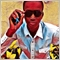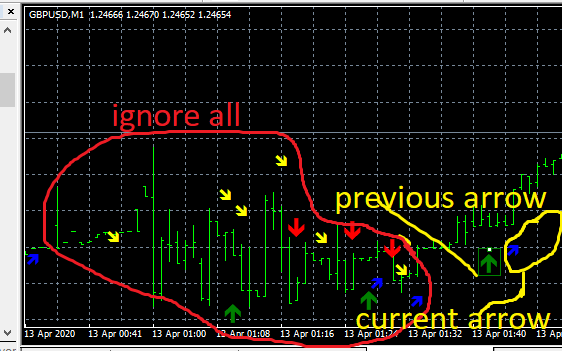# get the previous object on chart3213

hello.. please how to get the previous object on chart

this code not getting the previous object

``` for(int iObj=ObjectsTotal()-1; iObj >= 0; iObj--){
string on = ObjectName(iObj);
}  ```21724

Abubakar Saidu: how to get the previous object on chart — this code not getting the previous object
1. There are two types of arrows.
1. Buffers of type arrow. View (Alt+V) → Data Window (Control+D) If you see your "main indicator" and a value where there is an arrow, then read the buffer, (using iCustom) and do what you want with it.
Detailed explanation of iCustom - MQL4 programming forum
2. Otherwise, it is drawing arrow objects. Charts (Alt+C) → Objects → Objects List (Control+B).
Find the corresponding object and find the naming pattern. Then you can get all appropriate objects and their values.
Object Functions

2. Your code assumes objects. It is "getting the previous object." It is getting all objects (with the prefix "Arrowbuy".)
3. You need to also filter by the object's time, and keep only the latest two.3213

am i calling the previous object on chart correctly

```   static int last_i  = -1;
for(int i = ObjectsTotal(OBJ_ARROW)-1; i>= 0; i--)
{
string n = ObjectName(ChartID(), i, -1, OBJ_ARROW);
if ((int)ObjectGetInteger(ChartID(),n, OBJPROP_TIME)>0 && StringFind(n, "Arrow_Buy") == 0) conb=true;
}
```21724

Abubakar Saidu:am i calling the previous object on chart correctly

That's not what your code is doing.

1. It processes all arrows with the proper prefix.
2. When to you ever expect the arrow time to be zero?
3. You are still not "keep only the latest two."3213

ah.. am confused on how to arrange it perfect this is my first time handling toughly with Objects codes

```   void OnTick()
{
int total = ObjectsTotal(OBJ_ARROW);
//--
int i_last_sell = FindLastSellIndex(234);
string s_last_sell = (string)ObjectName(ChartID(), i_last_sell, -1, OBJ_ARROW);
datetime t_last_sell =(int) ObjectGetInteger(ChartID(), s_last_sell, OBJPROP_TIME);
double conb=false,cons=false;
for(int iObj=ObjectsTotal()-1; iObj >= 0; iObj--)
{
string on = ObjectName(iObj);
conb=true;
if (StringFind(on, "Arrow_sell") == 0)
cons=true;
}
{
}
if(sellsignal() && t_last_sell == 0 && cons==true)
{
}

}

{
static int offset = 0;
static int last_i  = -1;
for(int i = ObjectsTotal(OBJ_ARROW)-1; i>= 0; i--)
{
string n = ObjectName(ChartID(), i, -1, OBJ_ARROW);
int code = (int)ObjectGetInteger(ChartID(), n, OBJPROP_ARROWCODE);
if(code == cd)
{
offset = ObjectsTotal(OBJ_ARROW) - i;
//algo2 = true;
last_i = i;
return i;
}
}
return -1;
}

int FindLastSellIndex(int cd)
{
for(int i = ObjectsTotal(OBJ_ARROW)-1; i>= 0; i--)
{
string n = ObjectName(ChartID(), i, -1, OBJ_ARROW);
int code = (int)ObjectGetInteger(ChartID(), n, OBJPROP_ARROWCODE);
if(code == cd)
{
return i;
}
}
return -1;
}```3213

Hi.. could anyone help mw with the above code.. i cant get it work..

Thanks